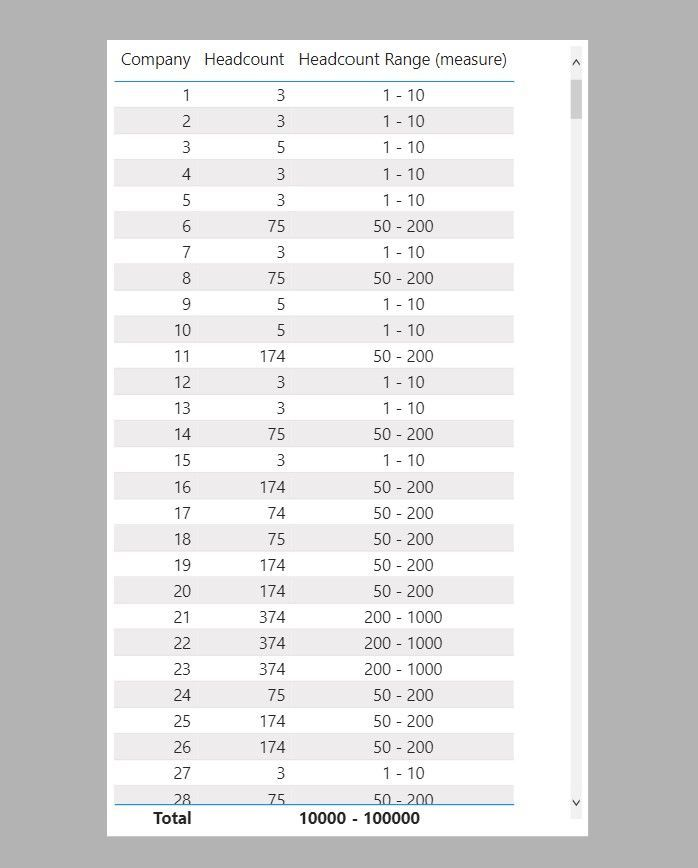cancel
Showing results for
Did you mean:Frequent Visitor

## Grouping Values by Ranges Retreived from a Different Table under Two Conditions

Dear Community,

I have the following two tables in my data model:Using a DAX-Expression, the column "Headcount Range" in the "Company List" table shall be filled with the "Range" value from the "Headcount Ranges" table, by applying the condtion that "Headcount" is greater or equal to "Lower Threshold" AND smaller or equal to "Upper Threshold".

Besides RELATED(), I have been experimenting with the LOOKUPVALUE() function, but there does not seem to be a way to formaulate the condition in the third argument, is there?.

Could sombedoy help me out with a solution, please?

Best regards,

Wolfi

1 ACCEPTED SOLUTIONSuper User

Thank you for the file. See if this works for you.

A. As a calculated column

``````Headcount Range =
VAR _Threshold =
CALCULATE (
MIN ( 'Headcount Ranges'[Upper Threshold] ),
FILTER (
)
) // Calculates the minimum upper threshold value in the Headcount table which is greater or equal to the Company list[Headcount]
RETURN
LOOKUPVALUE (
)
// Retruns the range value on the Headcount Ranges table where the upper threshold is the same as the calculated _Threshold value
``````B. As a measure

``````Headcount Range (measure) =
VAR _Threshold =
CALCULATE (
MIN ( 'Headcount Ranges'[Upper Threshold] ),
FILTER (
)
)
RETURN
LOOKUPVALUE (
)
``````Sample PBIX file attached

In doing so, you are also helping me. Thank you!

Proud to be a Super User!

4 REPLIES 4Frequent Visitor

Many thanks, Paul, for a job very well done! Your solution works just as it is supposed to!Super User

It would help if you shared some sample dummy data or a link to a dummy PBIX file

In doing so, you are also helping me. Thank you!

Proud to be a Super User!Frequent Visitor

Hi Paul,

Best,

WolfiSuper User

Thank you for the file. See if this works for you.

A. As a calculated column

``````Headcount Range =
VAR _Threshold =
CALCULATE (
MIN ( 'Headcount Ranges'[Upper Threshold] ),
FILTER (
)
) // Calculates the minimum upper threshold value in the Headcount table which is greater or equal to the Company list[Headcount]
RETURN
LOOKUPVALUE (
)
// Retruns the range value on the Headcount Ranges table where the upper threshold is the same as the calculated _Threshold value
``````B. As a measure

``````Headcount Range (measure) =
VAR _Threshold =
CALCULATE (
MIN ( 'Headcount Ranges'[Upper Threshold] ),
FILTER (
)
)
RETURN
LOOKUPVALUE (
)
``````Sample PBIX file attached

In doing so, you are also helping me. Thank you!

Proud to be a Super User!